# Make Your Numeric Division Faultless in Power Query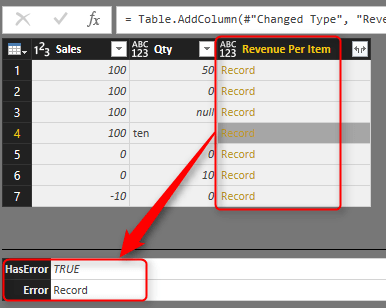When you work with data it is normal that you apply numeric calculations. Numeric calculations in Power Query depends on the nature of data returns different results. One of the most error prone calculations is division. Power Query behave differently when you divide a number by zero, zero by zero, number by null, and non-numeric values. One of the most frustrating facts is that not all of these calculations ends up to an error. So you can’t just remove error rows simply. In this post I’ll explain some examples of output for division and a method to find these rows.

## Sample Data Set

For this post I’ll use a sample excel file which has most of possible combinations that I might face in a division calculation. The table below is some records with Sales Amount and Quantity. and as a simple calculation I want to find out Revenue Per Item which would be result of [Sales]/[Quantity].In table above there are nulls, texts, zeros, negative, and positive values. Now let’s bring the table into Power Query (Excel or Power BI) and apply the division

## Simple Division Calculation

Here is the data set loaded into Power Query. As you can see Quantity column shows the data type as numeric and text.Now If I apply a simple division calculation as a new custom columnThe result would be as below;As you see the result set has different outputs depends on the inputs. if number is divided by a zero value result would be positive or negative infinity (depends on the number). if one of the values be null, then result set would be null. if zero divided by zero then result would be NaN! and in case of dividing a number by string or reverse there will be an error raised. Now Let’s look at each output separately.

# Error Output

Error in sample above happened when one of the values is not number. Fortunately errors can be simply found by TRY keyword. Here is how I change the calculation of Revenue Per Item:

`= try [Sales]/[Qty]`Result set this time would be a Record for each calculation.The record has two columns: HasError (which says does this record contains error or not), and Error Record (which would be the error happened in details). So I can add a custom column with a condition on HasError to see if record contains error or not. In expression below if I find an error I will return zero as the result.

`= if [Revenue Per Item][HasError] then 0 else [Revenue Per Item][Value]`The result set this time would be: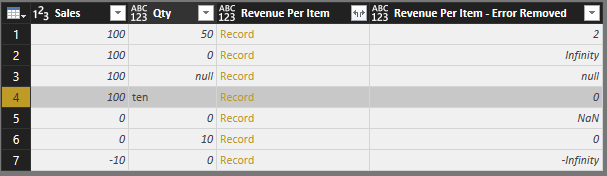In this example I just returned zero if I find error. but you can return error message if you like with [Revenue Per Item][ErrorMessage]. This method is great error handling method when an error out of blue happens in your data set. I always recommend using TRY method to get rid of errors that might stop the whole solution to work properly.

I have to mention that steps above are separated to show you how the output of try expression looks like. In fact you can combine both steps above in single step with TRY OTHERWISE as below (Thanks to Maxim Zelensky for pointing this out);

`= try [Sales]/[Qty] otherwise 0`

# Infinity

Error output can be handled with TRY. However Infinity and -Infinity are not errors! These are number values in Power Query, named Number.PositiveInfinity and Number.NegativeInfinity.• PositiveInfinity happens when a positive number divided by zero
• NegativeInfinity happens when a negative number divided by zero

You can’t find these with error handling because as I mentioned earlier these are not error values! You can however check these values to see if a value is NegativeInfinity or PositiveInfinity with sample code below:

```Pinfinity=(if x=Number.PositiveInfinity then false else true),
Ninfinity=(if x=Number.NegativeInfinity then false else true)
```

# NaN

NaN is another output which happens when zero is divided by zero. NaN is a number value like positive and negative infinity. So you can’t use error handling to spot them out of millions of records.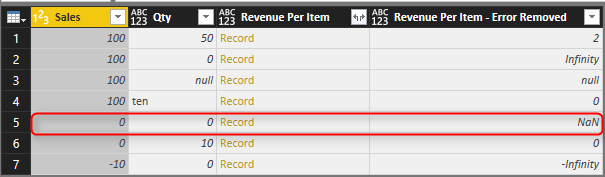You can find it with Number.IsNaN function which works as below;

`Nan=(if Number.IsNaN(x) then false else true)`

# Null Check

Null values always happens in the data, and best practice is always replace them with default values. In numeric calculations if a null value appears in one of the values the result of the calculation will be null.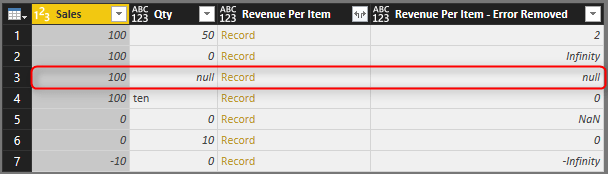You can simply find nulls with if condition such as below;

`Null=(if x=null then false else true)`

# Function to Check All Anomalies

Anomalies in outputs such as above examples happens in most of the cases, and I found it useful to have a function to check all these options. The function below checks Null, NaN, PositiveInfinity, and NegativeInfinity. It doesn’t check errors however. Error handling is best to be applied on the calculation level as we’ve done earlier in this post. Here is the code for the function:

```let
Source = (x as any) =>
let
Null=(if x=null then false else true),
Pinfinity=(if x=Number.PositiveInfinity then false else true),
Ninfinity=(if x=Number.NegativeInfinity then false else true),
Nan=(if Number.IsNaN(x) then false else true)
in
Null and Pinfinity and Ninfinity and Nan
in
Source```With function above, now I can add a custom column to my data set, and validate rows;Final result set shows me which record is validated and which one is not. Note that the row containing error has been handled previously so it is validating as true here.# Summary

Divide by zero is not the only error that happens in Power Query. Power Query returns different results such as Error, NaN, Positive Infinity, Negative Infinity, and Null. For a proper faultless calculation you have to consider all these exceptions. In this post You’ve learned how to spot these exceptions easily and make your calculation faultless with small amount of effort. If you like to learn more about Power Query and Power BI read Power BI online book; from Rookie to Rock Star.

Save

Save

Save

Save

Trainer, Consultant, Mentor
Reza Rad is a Microsoft Regional Director, an Author, Trainer, Speaker and Consultant. He has a BSc in Computer engineering; he has more than 20 years’ experience in data analysis, BI, databases, programming, and development mostly on Microsoft technologies. He is a Microsoft Data Platform MVP for nine continuous years (from 2011 till now) for his dedication in Microsoft BI. Reza is an active blogger and co-founder of RADACAD. Reza is also co-founder and co-organizer of Difinity conference in New Zealand.
His articles on different aspects of technologies, especially on MS BI, can be found on his blog: https://radacad.com/blog.
He wrote some books on MS SQL BI and also is writing some others, He was also an active member on online technical forums such as MSDN and Experts-Exchange, and was a moderator of MSDN SQL Server forums, and is an MCP, MCSE, and MCITP of BI. He is the leader of the New Zealand Business Intelligence users group. He is also the author of very popular book Power BI from Rookie to Rock Star, which is free with more than 1700 pages of content and the Power BI Pro Architecture published by Apress.
He is an International Speaker in Microsoft Ignite, Microsoft Business Applications Summit, Data Insight Summit, PASS Summit, SQL Saturday and SQL user groups. And He is a Microsoft Certified Trainer.
Reza’s passion is to help you find the best data solution, he is Data enthusiast.

## 13 thoughts on “Make Your Numeric Division Faultless in Power Query”

•Maxim Zelensky says:

Hi Reza, nice explanation!
But why don’t you use “try expression1 otherwise expression2” instead of “if then else”?

•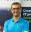Reza Rad says:

Hi Maxim,
try otherwise is much easier for sure as you mentioned. I just added that as two extra step to show what is the Error Record type of output when we work with try expression.
Will add a sentence to explain the easier method you’ve mentioned now.

Cheers,
Reza

•Maxim Zelensky says:

Agreed, missed this “explanatory” point. Well done!

•Ed Hansberry says:

Is there a circumstance where you would be better off using just TRY vs TRY-OTHERWISE?

It is interesting to see what is going on in the background with the two field record, but not sure I can see a practical real world example where I’d want to leave out Otherwise.

•Reza Rad says:

using try/otherwise would be simple way of doing that I’d say. If I want to check if there is an error then do a default. I’ll normally use that; try …. otherwise -1
using TRY with checking the record in details later on, is normally for more troubleshooting, and detailed investigation.
Cheers
Reza

•chris aaslid says:

Love the content, very helpful and logical. I had one question: in the NaN record in your last picture, it’s still showing as TRUE in the Validation Result. Shouldn’t it be saying FALSE like the others?

•Reza Rad says:

Hi Chris,

Awesome catch. I haven’t noticed that myself. Very good job pointing that out. For some reason comparison with Number.NaN is not working properly. I have changed it and used Number.IsNaN function instead. blog post is updated.
Thanks again

Cheers
Reza

•chakri says:

how do i divide in Direct query mode?

•Reza Rad says:

I DirectQuery mode I suggest to do all your calculation in the data source side
Cheers
Reza

•Yeliz says:

Hi Reza.. thanks so much for all the information you provide here.. I am new to BI so my question might sound a little stupid, sorry for that..
Why do we not replace Error with 0 simply rather than using TRY?

•Reza Rad says:

not a stupid question at all.
in your code, you should have a mechanism to find out that error happened (without your code stopping execution and breaking). That is why we use TRY, to find out that an error happened. our code continues execution, and it doesn’t fail, then we can take an action based on the error happened.
Cheers
‘Reza

•Sophie says:

Great tips- thanks!